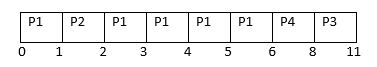Skip to content
Related Articles
GATE | GATE CS Mock 2018 | Question 16
• Last Updated : 10 Jan, 2018

Consider the following CPU processes with arrival times (in milliseconds) and length of CPU bursts (in milliseconds) except for process P4 as given below:

```Process Arrival Time   Burst Time
P1       0              5
P2       1              1
P3       3              3
P4       4              x
```

If the average waiting time across all processes is 2 milliseconds and pre-emptive shortest remaining time first scheduling algorithm is used to schedule the processes, then find the value of x ?
(A) 1
(B) 2
(C) 4
(D) None of these

Answer: (B)

Explanation: If we take value of x is 2, then we have gantt chart asSo, completion time of P1, P2, P3, and P4 are 6, 2, 11, and 8 respectively.
Turn around time of P1, P2, P3, and P4 are 6, 1, 8, and 4 respectively.
Waiting time of P1, P2, P3, and P4 are 1, 0, 5, and 2 resectively.
Therefore, average waiting time = (1+0+5+2) / 4 = 8/2 = 2

Option (B) is correct.

Quiz of this Question

Attention reader! Don’t stop learning now. Learn all GATE CS concepts with Free Live Classes on our youtube channel.

My Personal Notes arrow_drop_up